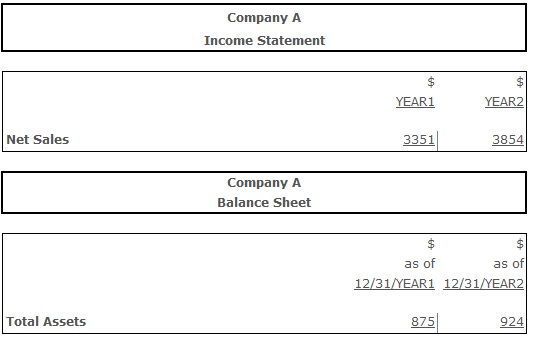# Total Asset Turnover

Total Asset Turnover – an activity ratio measuring the ability of a firm to effectively use its assets for the generation of sales. It can be calculated by dividing the net sales by average total assets. Unlike the fixed asset turnover, including only property, plant and equipment to calculation, this ratio measures how efficiently company uses all of its assets. The total asset turnover value measures how much goods and services are sold per every dollar of used assets during the analyzed period.

The normative range for total asset turnover values highly depends on the industry. Most commonly higher ratios indicate that less money is needed to invest for the sales generation. It is better to compare asset turnover ratio of a firm to industry averages, or to the firm's values of this ratio over past periods. Lower ratios usually indicate too heavy investments in assets or notable decline in sales. However, sometimes low asset turnover does not necessarily indicate negative trends for a firm. For example, when the modernization of a plant or mass replacement of the old equipment are being made. This means the high average amount of assets for one certain period, but generates positive results in future. Lower ratios may also indicate that the firm's assets aren't being used to their full capacity.

To make proper conclusions on the firm's asset turnover efficiency the following should be taken into account. First of all, the dynamics of the indicator values. Positive is the increasing trend of the indicator during the analyzed period. Secondly, it is reasonable to compare the total asset turnover value with competitors. If the company's value of this indicator will be greater than competitors', this would mean that the firm is more efficient in usage of its limited resources.

## Resolving the problems with the total asset turnover exceeding the normative range:

If the indicator value is not high enough, the optimization of the assets amount should be considered. This can be done through selling a part of underused out of circulation assets (in case increasing the use of assets is not planned), decreasing the stock (if their volume is excessive), taking active measures for the accounts receivables collection, etc. Measures for the company’s income increase also have positive effect on the asset turnover.

## Formula(s):

Total Asset Turnover = Net Sales ÷ Average Total Assets

Should be mentioned that for more precise calculation of this indicator the analyst should have an access to the internal company report. Average total assets amount is calculated as total assets at the end of the working day divided by the number of working days. If this data is missing, the value of assets at the end of the month can be taken and divided by twelve instead. Finally, if the analyst only possess the yearly information, he can calculate the average value of assets at the beginning and at the end of the year.

## Example:Total Asset Turnover (Year 1) = 3351 ÷ 875 = 3,82

Total Asset Turnover (Year 2) = 3854 ÷ 924 = 4,17

The increase of the total asset turnover from 3,82 in year 1 to 4,17 in year 2 is a good trend, because it indicates that the firm became able to generate more sales through its assets. In other words, in year 1 firm was generating 3,82 times as much sales as its assets, in year 2 it became generating 4,17 times as much sales as its assets.

## Conclusion:

Total asset turnover measures how efficiently assets are being used by a company for generation of sales. Commonly higher ratios are preferable, because they indicate the efficient usage of company's assets, but lower ratios not always mean negative trends for a company.